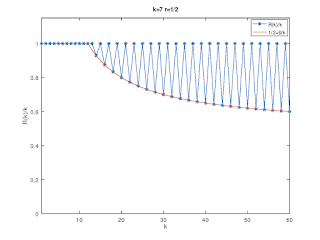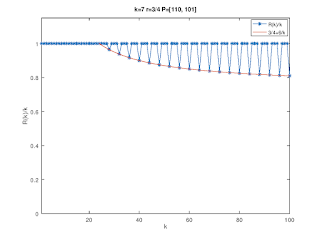## Friday, May 10, 2019

### Blind detection of convolutional codes

This post continues the topic started in this blog post, i.e., the detection of the presence of convolutional coding in a given bit stream.

The method outlined below is not new and a good reference it is this paper: M. Marazin, R. Gautier, G. Burel “Blind recovery of k/n rate convolutional encoders in a noisy environment

The basic idea is to arrange a given bit stream $$b_i$$ in matrices $$R_k$$ \begin{equation} B_k = \begin{pmatrix} b_0 & b_1 & b_2 & \cdots & b_{k-1}\\ b_k & b_{k+1} & b_{k+2} & \cdots & b_{2k-1}\\ \vdots & \vdots & \vdots & \ddots & \vdots \end{pmatrix} \end{equation} and then to determine the rank of $$R_k = \mathsf{rank}(B_k)$$ as a function of $$k$$. Note that for computing the rank $$B_k$$ is treated as a matrix with values in $$\mathbb{F}_2$$.

If the bit stream is completely random, all matrices $$B_k$$ will likely have rank $$R_k=k$$. However, the redundancy introduced by convolutional coding shows up as some matrices not having full rank, i.e., there are dependent rows in some $$B_k$$ and therefore $$R_k < k$$ for some $$k$$.

Starting from a $$k=7,\;r=1/2$$ mother code, rank deficiency plots are shown below for different puncturing patterns, together with the expected behavior.

Note that this method detects any redundancy (error coding) in a given bit stream and therefore can be generalized to other coding schemes.Rank deficiencies for the k=7 r=1/2 mother code.Rank deficiencies for a k=7 r=2/3 code obtained by puncturing.Rank deficiencies for a k=7 r=3/4 code obtained by puncturing.Rank deficiencies for a k=7 r=4/5 code obtained by puncturing.